# Percent of Change Lesson Plan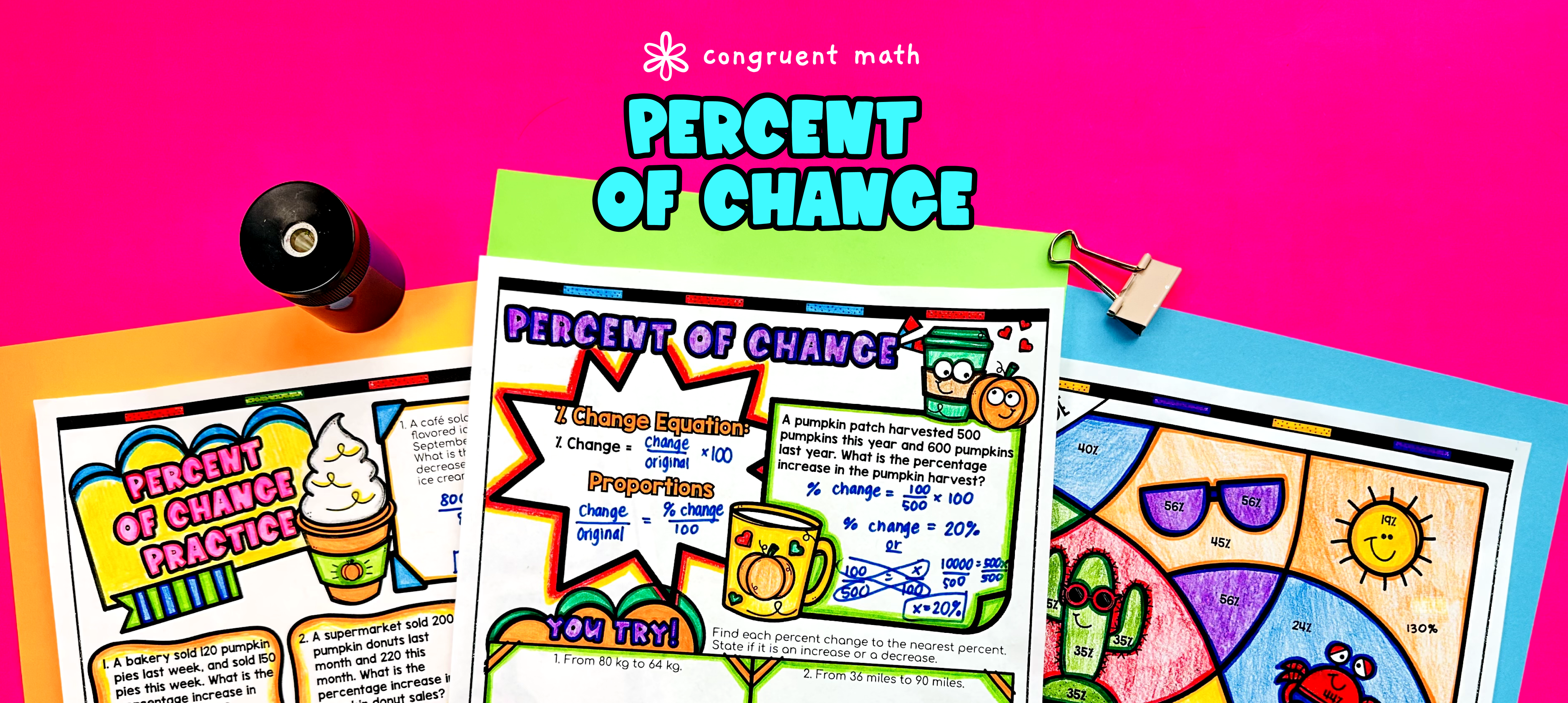Pin This

## Overview

Ever wondered how to teach percent increase and decrease in an engaging way to your seventh grade students?

In this lesson plan, students will learn about percent of change and its real-life applications. Through artistic, interactive guided notes, check for understanding, a doodle & color by number activity, and a maze worksheet, students will gain a comprehensive understanding of calculating percent change using the percent change formula as well as using proportions.

The lesson culminates with a real-life example that explores how percent of change can be used to solve word problems in practical situations. Students will read and write about real-life uses of percent change, further solidifying their understanding of the concept.

## Get the Lesson Materials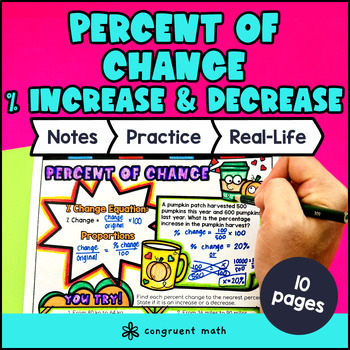\$4.25

## Learning Objectives

After this lesson, students will be able to:

• Calculate percent increase and decrease using the percent change formula

• Solve for percent change using proportions

• Apply the concept of percent change to real-life situations and word problems

## Prerequisites

Before this lesson, students should be familiar with:

• Basic understanding of fractions, decimals, and percents

• Knowledge of basic operations of integers (addition, subtraction, multiplication, and division)

• Understanding of how to substitute to solve an equation

## Key Vocabulary

• Percent increase

• Percent decrease

• Percent change

• Proportions

## Procedure

### Introduction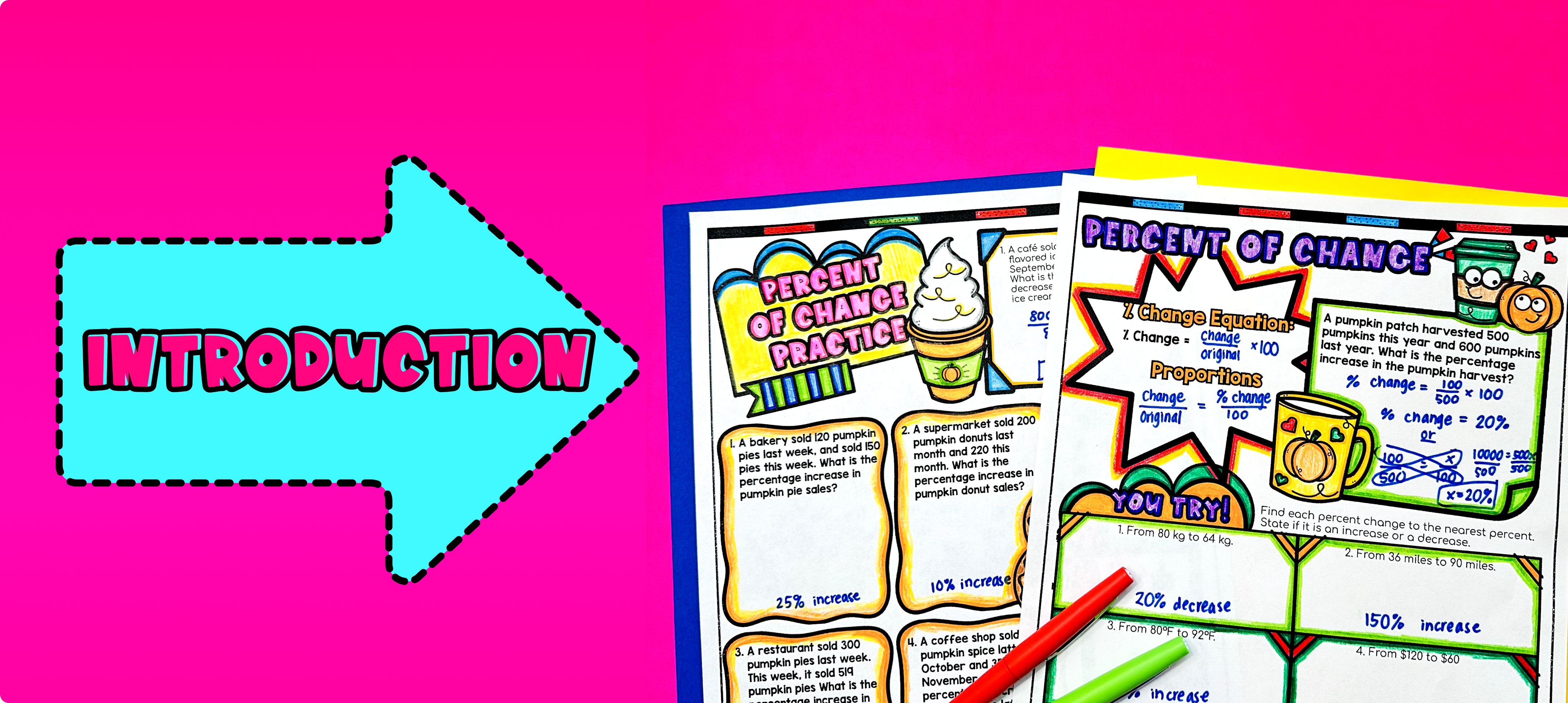Pin This

As a hook, ask students why understanding percent increase and decrease is important in real life situations. Refer to the last page of the guided notes for a detailed example as well as the FAQs below for ideas.

Use the first page of the guided notes to introduce the concept of percent change and how to calculate it using the percent change formula. Walk through the key points of the topic, including the steps to calculate percent change using the formula and the meaning of positive and negative percent change. Also introduce how you can set up proportions instead of using the percent change formula.

Based on student responses, reteach any concepts that students need extra help with. If your class has a wide range of proficiency levels, you can pull out students for reteaching, and have more advanced students begin work on the practice exercises.

### Practice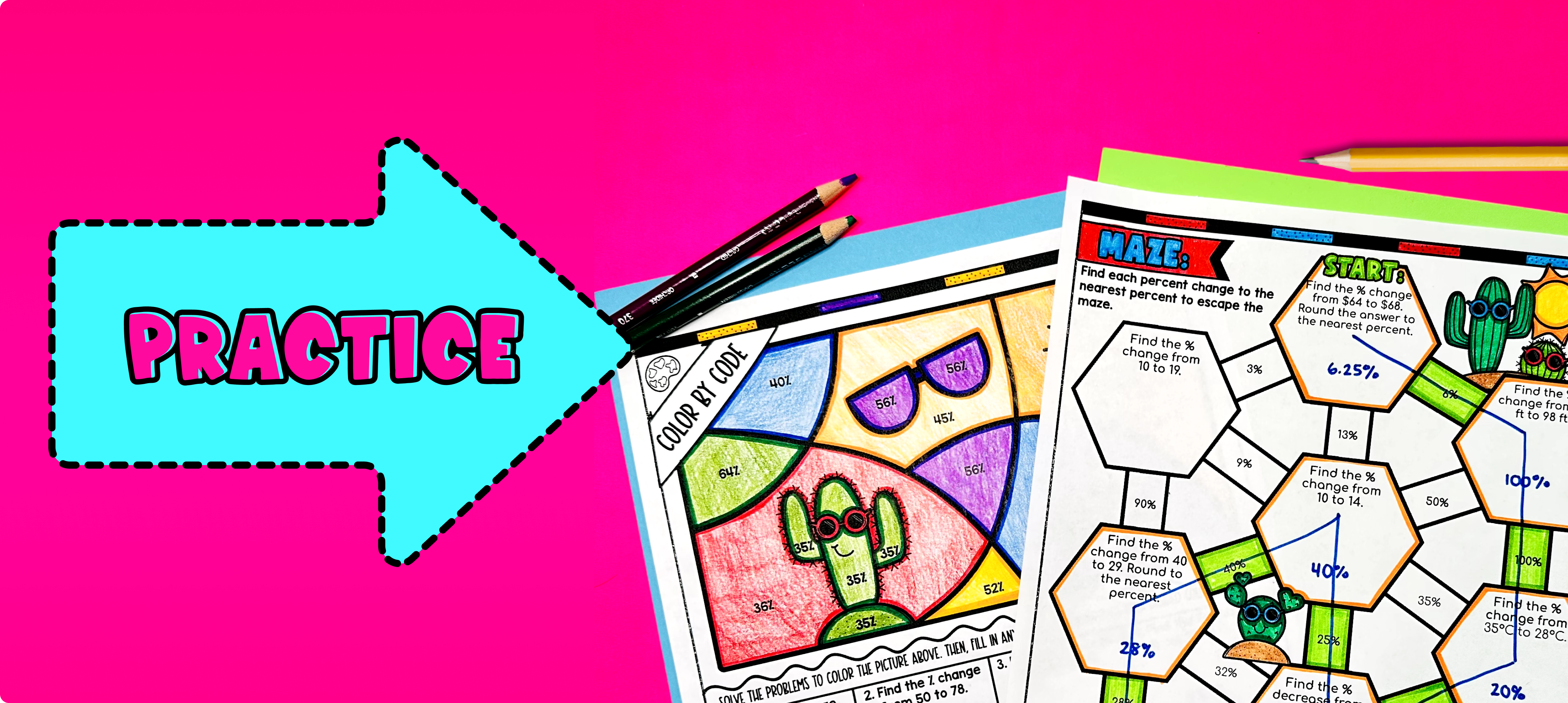Pin This

Have students practice calculating percent change using the Percent Change Formula and Percent Proportions using the practice worksheet (page 2 of guided notes) provided in the resource. Walk around the classroom to answer any student questions and provide support as needed.

Fast finishers can dive into the maze activity (page 3) or color by number activity (page 4) included in the resource. You can also assign it as homework for the remainder of the class.

### Real-Life Application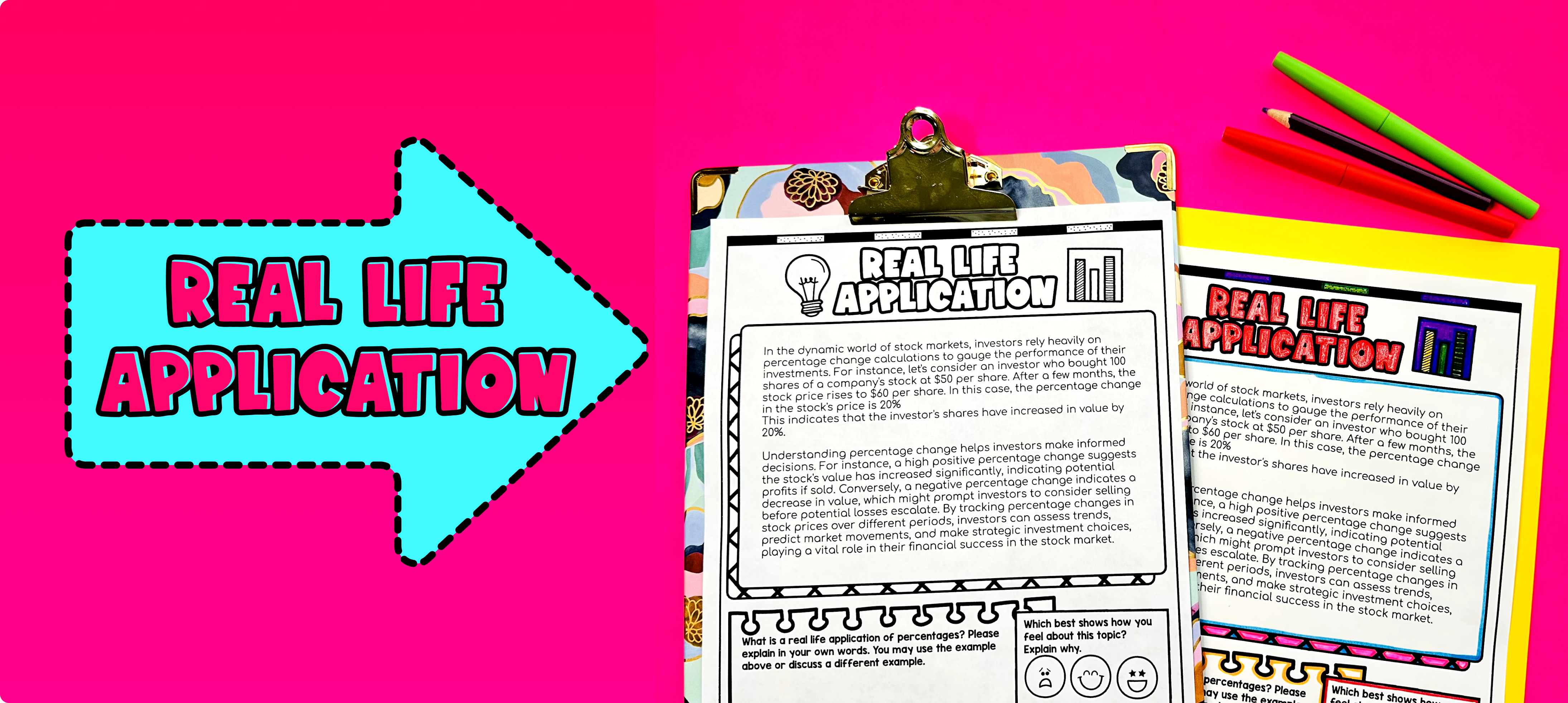Pin This

Bring the class back together, and introduce the concept of real-life applications of percent change. Explain to the students that percent change is a mathematical concept that is used in various real-world scenarios.

One example of a real-life application of percent change is in the field of finance and investments. Explain that when people invest their money in stocks or other financial instruments, the value of their investments can increase or decrease over time. This change in value can be measured using percent change. For example, if someone invests \$1,000 and their investment grows to \$1,200, the percent change would be (1200 - 1000) / 1000 = 0.2 or 20%. This means that their investment has grown by 20%.

Another example of a real-life application is in the context of sales and discounts. Explain that when stores offer discounts on their products, they often advertise the percent decrease in price. This helps consumers understand how much they can save on a purchase. For example, if a pair of shoes is originally priced at \$100 and is on sale for 20% off, the new price would be \$100 - (20% * \$100) = \$80. This means that the price of the shoes has decreased by 20%.

Ask for student volunteers to share any other real-life scenarios where percent change might be used. Encourage discussion and provide feedback on their ideas. Refer to the FAQ section in the teaching resource for more ideas and examples on how to teach the real-life application of percent change.

## Extensions

If you’re looking for digital practice for percent of change, try my Pixel Art activities in Google Sheets. Every answer is automatically checked, and correct answers unlock parts of a mystery picture. It’s incredibly fun, and a powerful tool for differentiation.

Here are 2 activities to explore:

A fun, no-prep way to practice percent of change is Doodle Math — they’re a fresh take on color by number or color by code. It includes multiple levels of practice, perfect for a review day or sub plan.

Here is an activity to try:

### Real-Life Math Project

A fun way to wrap this lesson with your students is with one of my real-life math projects. They enable students to see the application of math in an engaging activity where they play the role of an airline intern, using percentage to solve real world problems.

## Want more ideas and freebies?

Get my free resource library with digital & print activities—plus tips over email.